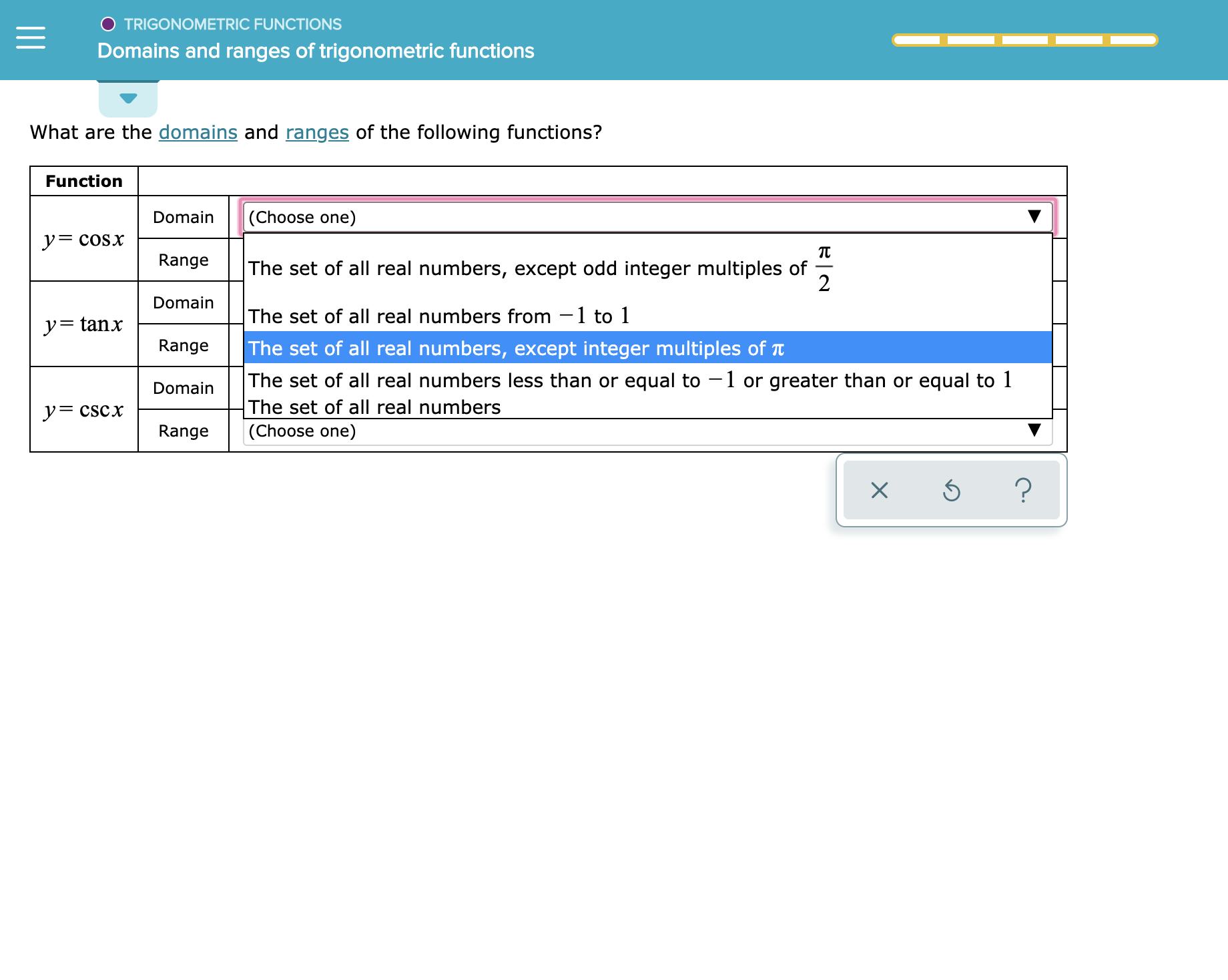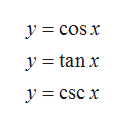O TRIGONOMETRIC FUNCTIONSDomains and ranges of trigonometric functionsWhat are the domains and ranges of the following functions?Function(Choose one)DomainycoSxRangeThe set of all real numbers, except odd integer multiples of2DomainThe set of all real numbers from -1 to 1y= tanxThe set of all real numbers, except integer multiples of TtThe set of all real numbers less than or equal to 1 or greater than or equal to 1RangeDomainThe set of all real numbersyCSCx|(Choose one)Range?

Question

See attachmenthelp_outlineImage TranscriptioncloseO TRIGONOMETRIC FUNCTIONS Domains and ranges of trigonometric functions What are the domains and ranges of the following functions? Function (Choose one) Domain ycoSx Range The set of all real numbers, except odd integer multiples of 2 Domain The set of all real numbers from -1 to 1 y= tanx The set of all real numbers, except integer multiples of Tt The set of all real numbers less than or equal to 1 or greater than or equal to 1 Range Domain The set of all real numbers yCSCx |(Choose one) Range ? fullscreen
Step 1

To find the domains and ranges of the following functions.help_outlineImage Transcriptionclose= cos x y tanx y csc x fullscreen
Step 2

Domain of a function is a set of all possible values of x (independent variable) that gives real values of y (dependent variable).

Range is the all these possible values of the y (dependent variable) which results after the substitution of x in the function.

Step 3

Y = cos x

Since the trigonometric function cosine is define for all the real values of x and give the resultant value in the range of -1 to 1...

Want to see the full answer?

See Solution

Want to see this answer and more?

Our solutions are written by experts, many with advanced degrees, and available 24/7

See Solution
Tagged in

Functions